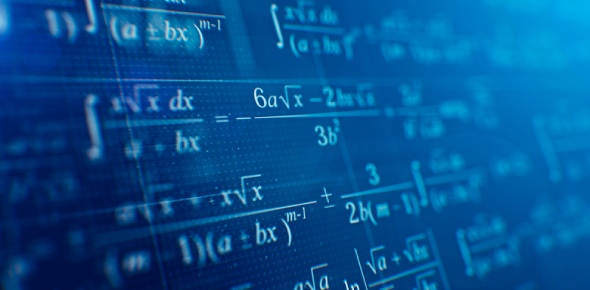# Mathematics Questions

10 Questions | Total Attempts: 5536SettingsWelcome to a quiz on a number of different subjects all under the umbrella of mathematics! In this one, we’ll be offering you a helpful quiz as used in online testing, in which we’ll check to see if your knowledge on numbers is up to scratch! Whether you need it for college or work, or you just want to broaden your knowledge on a relatively important subject, this quiz should satisfy your needs.

• 1.
Which of the following pairs have two numbers reciprocal to each other?   (I)     1/31 and – 31 (II)    SQRT2 and (SQRT2)/2 (III)    1/x and [(xy)1/2y]2
• A.

I & II

• B.

I, II & III

• C.

II & III

• D.

I Only

• E.

II Only

• 2.
If John were to add 4 gallons of water to a tank that is already 2/3 full of water, the tank would be 6/7 full.  How many gallons of water would the tank hold if it were half full?
• A.

11.5

• B.

10.5

• C.

10

• D.

21

• E.

11

• 3.
Is (1/x) >1?   (1)    x <1 (2)    x >1
• A.

Statement (1) ALONE is sufficient, but statement (2) alone is not sufficient.

• B.

Statement (2) ALONE is sufficient, but statement (1) alone is not sufficient.

• C.

BOTH statements TOGETHER are sufficient, but NEITHER statement ALONE is sufficient.

• D.

EACH statement ALONE is sufficient

• E.

Statements (1) and (2) TOGETHER are NOT sufficient

• 4.
Find the value of [(x2/ y2) Cubic Root(y3/x3 )]     (1)   x & y are integers      (2)  x & y are together either > 0 or <0
• A.

Statement (1) ALONE is sufficient, but statement (2) alone is not sufficient

• B.

Statement (2) ALONE is sufficient, but statement (1) alone is not sufficient

• C.

BOTH statements TOGETHER are sufficient, but NEITHER statement ALONE is sufficient

• D.

EACH statement ALONE is sufficient

• E.

Statements (1) and (2) TOGETHER are NOT sufficient

• 5.
[{10 SQRT(10) + 5 SQRT(5)} {10 SQRT(10) – 5 SQRT(5)}] =
• A.

1125

• B.

875

• C.

75

• D.

125

• E.

775

• 6.
68% of 781 - ?% of 289 = 389.47
• A.

39%

• B.

49%

• C.

43%

• D.

38%

• E.

51%

• 7.
A function f is defined for each positive four-digit integer m as f(m ) = 2x3y5z7w , where x, y, z and w are the thousands,hundreds, tens, and units digits of m, respectively.  If n and p are four-digit positive integers such that f(n)=125f(p), them n-p=?
• A.

30

• B.

9

• C.

18

• D.

20

• E.

10

• 8.
What is the value of the ratio of a2   to b3   (1)   the ratio of a3 to b is 2/7 (2)   the ratio of b2 to 1 is 49/4
• A.

Statement (1) ALONE is sufficient, but statement (2) alone is not sufficient

• B.

Statement (2) ALONE is sufficient, but statement (1) alone is not sufficient

• C.

BOTH statements TOGETHER are sufficient, but NEITHER statement ALONE is sufficient

• D.

EACH statement ALONE is sufficient

• E.

Statements (1) and (2) TOGETHER are NOT sufficient

• 9.
Is one of the angles of quadrilateral PQRS equal to 120 degrees? (1)   Two of the angles of PQRS namely angle P and angle R are right angles. (2)   The degree measure of angle Q is twice the degree measure of angle S.
• A.

Statement (1) ALONE is sufficient, but statement (2) alone is not sufficient.

• B.

Statement (2) ALONE is sufficient, but statement (1) alone is not sufficient

• C.

BOTH statements TOGETHER are sufficient, but NEITHER statement ALONE is sufficient

• D.

EACH statement ALONE is sufficient

• E.

Statements (1) and (2) TOGETHER are NOT sufficient

• 10.
The product of three consecutive odd numbers is 35805. What is the smallest of three numbers?
• A.

31

• B.

41

• C.

43

• D.

33

• E.

37

Related Topics